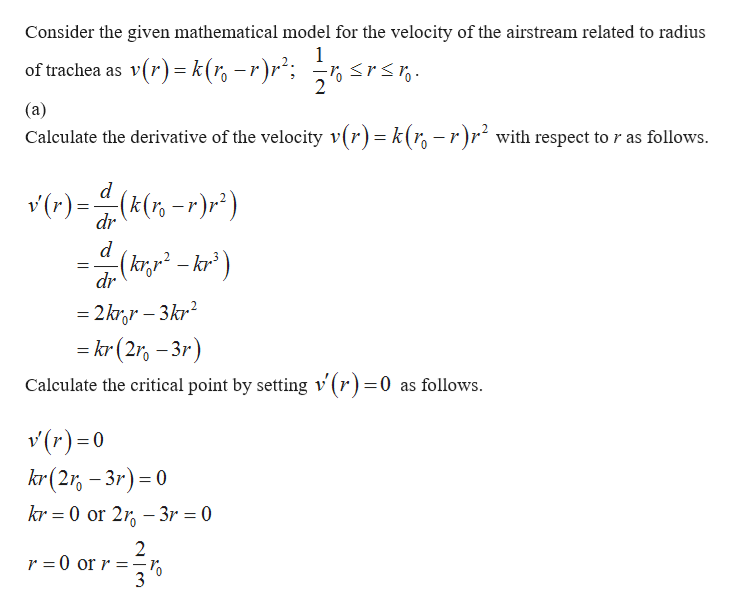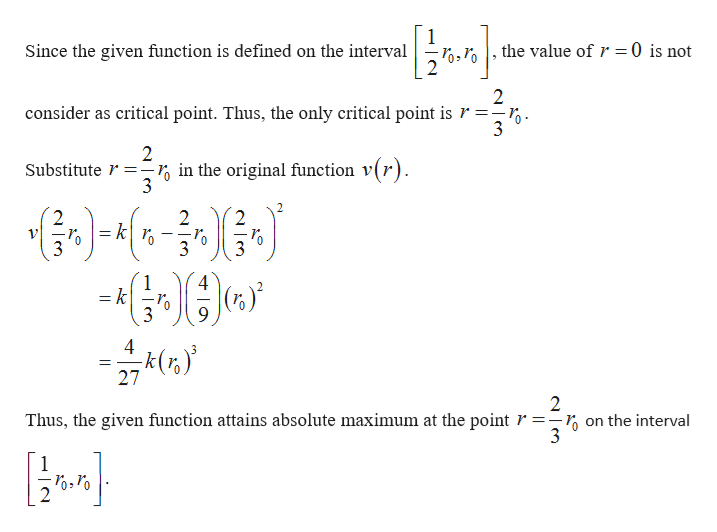# When a foreign object lodged in the trachea (windpipe) forces a person to cough, the diaphragm thrusts upward causing an increase in pressure in the lungs. This is accompanied by a contraction of the trachea, making a narrower channel for the expelled air to flow through. For a given amount of air to escape in a fixed time, it must move faster through the narrower channel than the wider one. The greater the velocity of the airstream, the greater the force on the foreign object. X rays show that the radius of the circular tracheal tube contracts to about two-thirds of its normal radius during a cough. According to a mathematical model of coughing, the velocity v of the airstream is related to the radius r of the trachea by the equationv(r) = k(r0 − r)r2    12r0 ≤ r ≤ r0where k is a constant and r0 is the normal radius of the trachea. The restriction on r is due to the fact that the trachea wall stiffens under pressure and a contraction greater than12r0is prevented (otherwise the person would suffocate).(a) Determine the value of r in the intervalat which v has an absolute maximum.  (b) What is the absolute maximum value of v on the interval?

Question

When a foreign object lodged in the trachea (windpipe) forces a person to cough, the diaphragm thrusts upward causing an increase in pressure in the lungs. This is accompanied by a contraction of the trachea, making a narrower channel for the expelled air to flow through. For a given amount of air to escape in a fixed time, it must move faster through the narrower channel than the wider one. The greater the velocity of the airstream, the greater the force on the foreign object. X rays show that the radius of the circular tracheal tube contracts to about two-thirds of its normal radius during a cough. According to a mathematical model of coughing, the velocity v of the airstream is related to the radius r of the trachea by the equation

v(r) = k(r0 − r)r2
 1 2
r0 ≤ r ≤ r0

where k is a constant and r0 is the normal radius of the trachea. The restriction on r is due to the fact that the trachea wall stiffens under pressure and a contraction greater than

 1 2
r0

is prevented (otherwise the person would suffocate).

(a) Determine the value of r in the interval
 at which v has an absolute maximum.

(b) What is the absolute maximum value of v on the interval?

check_circle

Step 1help_outlineImage TranscriptioncloseConsider the given mathematical model for the velocity of the airstream related to radius 1 v(r)k(-r)r of trachea as 2 (a) Calculate the derivative of the velocity v(r) = k(r, -r)r with respect to r as follows. d r)=(k(r)r) dr kr dr 2krr-3kr2 kor (2-3r) Calculate the critical point by setting v (r)=0 as follows. v(r)0 kr (23r) 0 kr 0 or 23r 0 2 r =0 or r =- fullscreen
Step 2help_outlineImage Transcriptionclosethe value of r = 0 is not Since the given function is defined on the interval 2 2 consider as critical point. Thus, the only critical point is r 2 in the original function v(r). Substitute =- fr)-4 2 = k 2 2 -0 3 3 1 = k| 4 k(r 27 2 Thus, the given function attains absolute maximum at the point r =-, on the interval 0 1 fullscreen

### Want to see the full answer?

See Solution

#### Want to see this answer and more?

Solutions are written by subject experts who are available 24/7. Questions are typically answered within 1 hour.*

See Solution
*Response times may vary by subject and question.
Tagged in

### Other